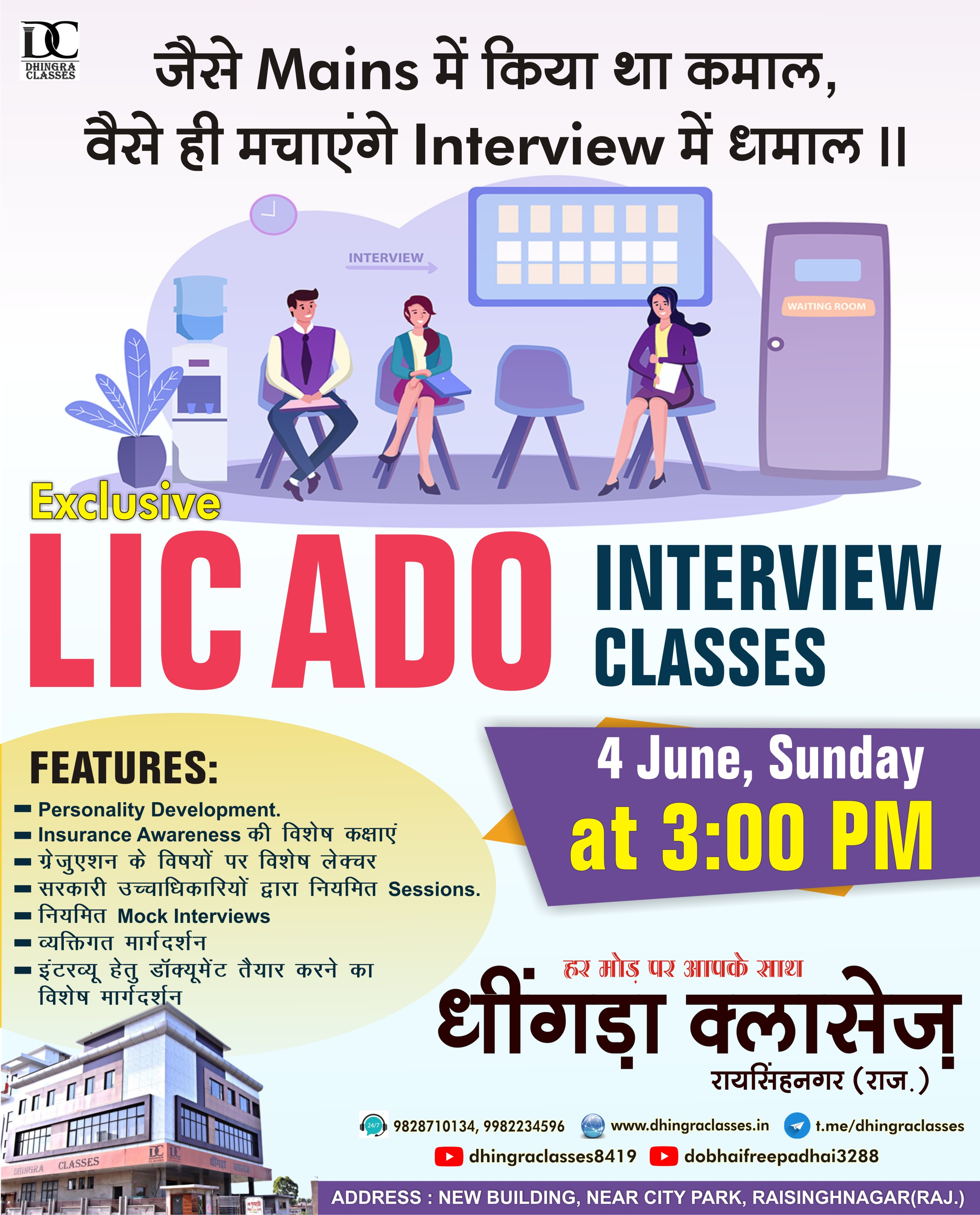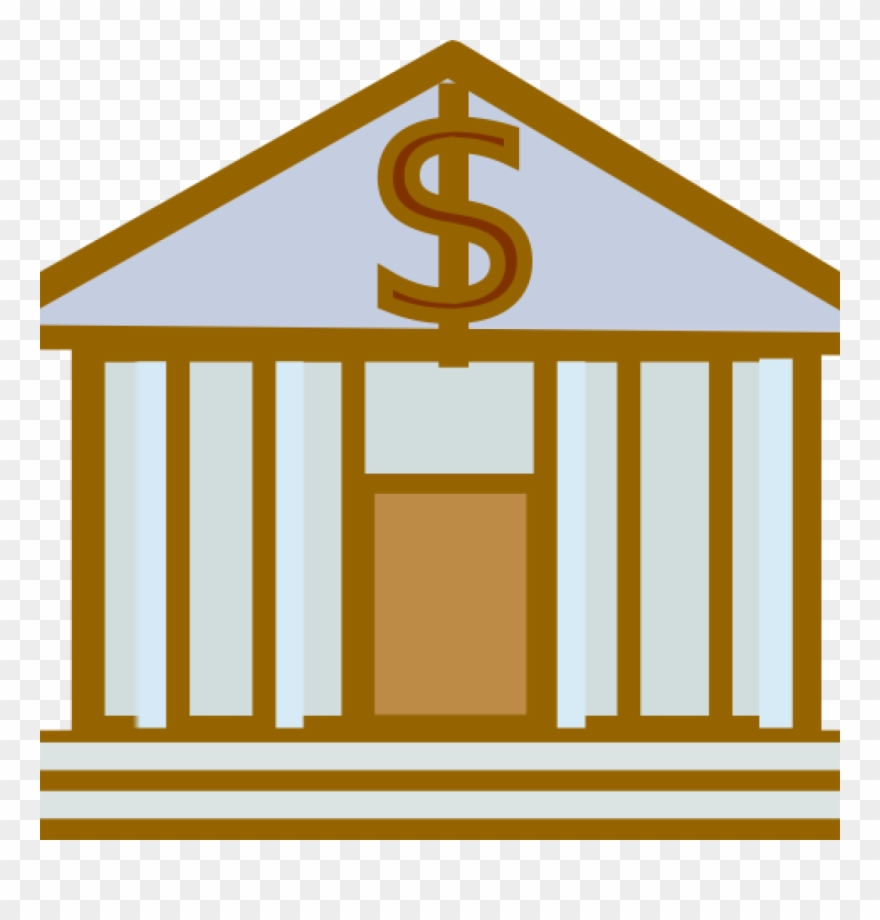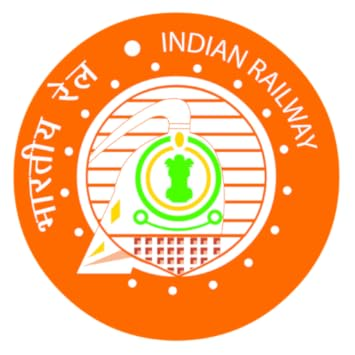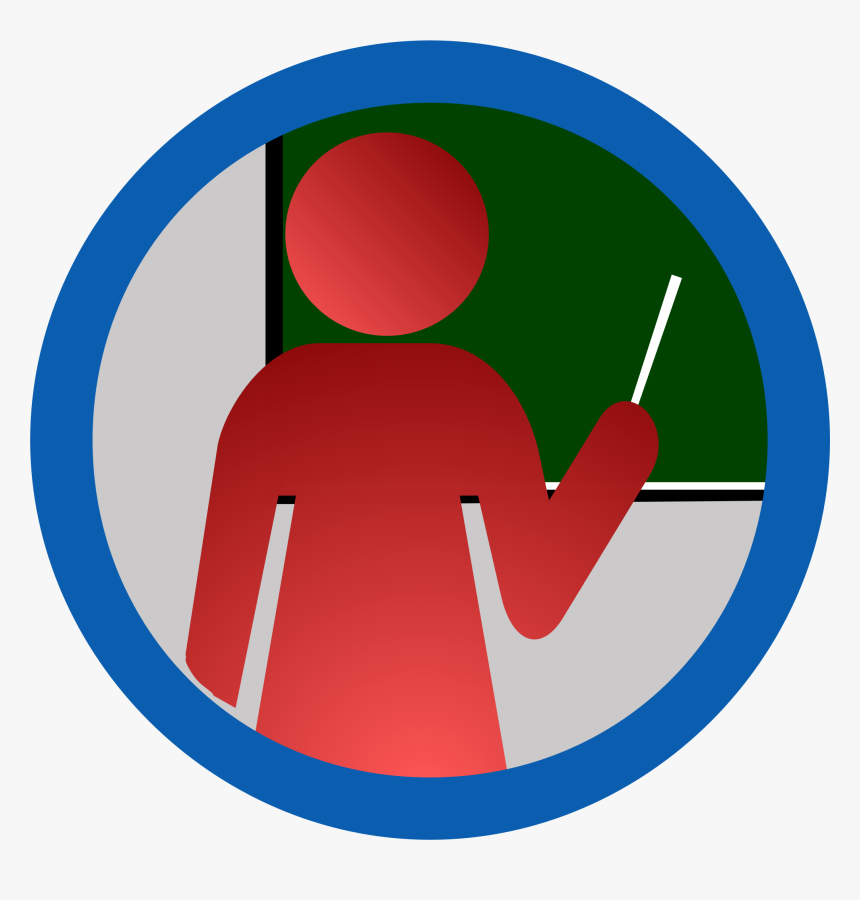### SBI Clerk Pre Asked Questions 1st Shift:

1).
1. x2 – 5x + 6 = 0
2. y2 – 9y + 20 = 0
2).
1. 6x2 – 11x + 4 =0
2. 50y2 – 25y + 3 =0
3).
1. x2 – 4x – 12 =0
2. y2 – 5y – 14 =0
4).

1. 3x2 + 8x + 4=0
2. 6y2 + 7y + 2 =0

Spotting Errors:
When at last I succeeded in convincing(1) him that I want to get home(2) quickly he agreed to (3)give me a lift in his car(4)N.E (5)

An Army officer pointed out that overall(1) the new matrix system would worsened(2) the disparity between(3) military officers and their civilian counterparts.(4)N.E
(5)

The new mining law (1)requires all blocks with (2)identified mineral reserves to  auction(3) instead of allotted administratively(4)N.E
(5)

Simplification:

48% of 4800 + 24% of 1260 =?
76.36 + 2984.317 + 39465.11 =?
2508 ÷ 15.02 + ? × 11 = 200

Number series:

1.  1, 244, 163, 190, 181, ?
2.  250, ?, 190, 167, 148, 131
3.  18, 8, 6, 9, 23, ?
4.  36, 18, 6, 3, 1, ?

5.  18, 29, 42, 53, ?, 77

Direction: Study the following Pie-chart carefully to answer these questions.
Total Students = 65001. What is the value of half of the difference between the number of students in MBA and MBBS?
2. How much more percentage (approximately) of students are in MBA as compared to students in B.Ed.?
3. What is the total number of students in B.Ed., Pharmacy and MBBS together?
4. What is the respective ratio between the number of students in Pharmacy and the number of students in B.Tech?

Coding decoding:
In a certain code language,
‘economics is not money’ is coded as ‘ka la ho ga’
‘Demand and supply economics’ is coded as ‘mo ta pa ka’
‘Money makes only part’ is coded as ‘zi la ne ki’
‘Demand makes supply economics’ is coded as ‘zi mo ka ta’
1. What may be the possible code for ‘demand only more’ in the given code?
2. What may be the possible code for work and money’ in the given code language?
3. What is the code for ‘makes’ in the code language?
4. What is the code for ‘money’ in this code language?

## TOP COURSES

Courses offered by Us### BANKING

SBI/IBPS/RRB PO,Clerk,SO level Exams### SSC

WBSSC/CHSL/CGL /CPO/MTS etc..### RAILWAYS

NTPC/GROUP D/ ALP/JE etc..### TEACHING

REET/Super TET/ UTET/CTET/KVS /NVS etc..